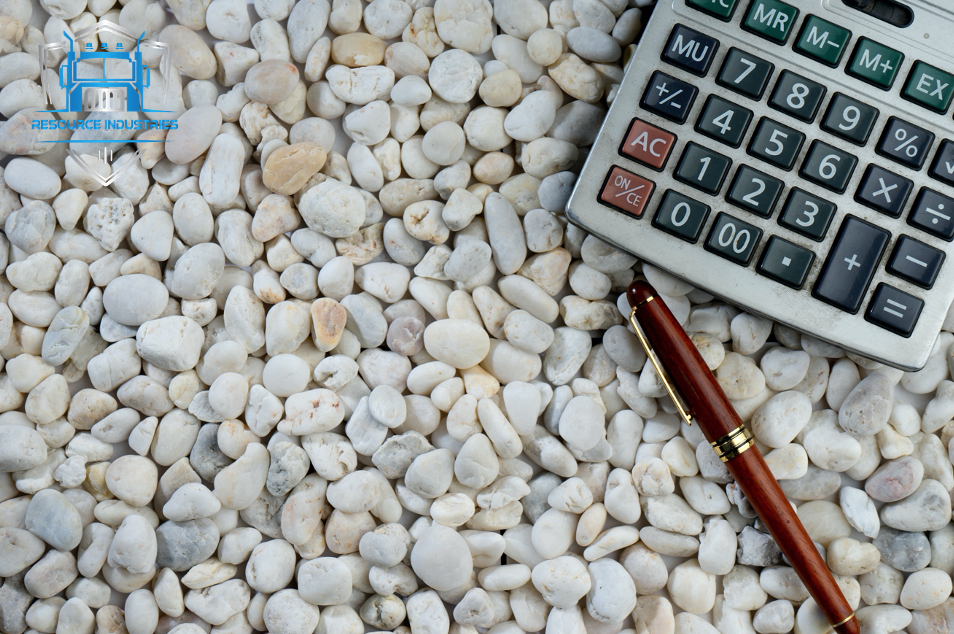Calculating the amount of aggregate materials you'll need for a construction project is an important step in the planning process. Aggregate materials, such as gravel, sand, and crushed stone, are used in a variety of applications, including concrete production, road construction, and landscaping. Accurate calculations can help ensure that you have enough materials on hand to complete the project and avoid delays due to shortages.

There are several formulas that can be used to calculate the amount of aggregate materials you'll need. One of the most commonly used formulas is the material requirement calculation. To use this formula, you'll need to know the length, width, and depth of the area where the aggregate will be placed.

To calculate the amount of aggregate you'll need in cubic yards, use the following formula:

(Length (ft) x Width (ft) x Depth (ft)) / 27 = Cubic Yardsage

For example, if you need to cover an area that is 20 feet long, 10 feet wide, and 4 inches deep with crushed stone, the calculation would be:

(20 x 10 x 0.33) / 27 = 2.22 cubic yards

Another formula that can be used to calculate the amount of aggregate materials you'll need is the volume calculation. This formula is often used when working with irregularly shaped areas or when the depth of the aggregate varies. To use this formula, you'll need to divide the area into smaller sections and calculate the volume of each section separately.

To calculate the volume of an irregularly shaped area in cubic yards, use the following formula:

Volume (cubic yards) = Width (ft) x Length (ft) x Depth (ft) / 27

For example, if you need to cover an area that is 20 feet long, 10 feet wide, and 4 inches deep with gravel, the calculation would be:

Volume (cubic yards) = 20 x 10 x 0.33 / 27 = 2.22 cubic yards

It's important to note that these formulas are based on the assumption that the aggregate materials will be compacted to a specific density. In order to ensure that you have enough materials for your project, it's recommended that you add an additional 10-20% to your calculations to account for any losses during transportation and compaction.

In conclusion, calculating the amount of aggregate materials you'll need for a construction project is an important step in the planning process. By using the material requirement or volume calculation formulas, you can accurately determine the amount of materials you'll need to complete your project. Remember to add a cushion to your calculations to account for any losses during transportation and compaction.